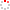CIOMP OpenIR  > 中科院长春光机所知识产出
 Propagation analysis of phase-induced amplitude apodization optics based on boundary wave diffraction theory Wang, W.; X. Zhang; Q. Y. Meng and Y. T. Zheng 2017 发表期刊 Optics Express卷号 25期号:21 摘要 Phase-induced amplitude apodization (PIAA) is a promising technique in high contrast coronagraphs due to the characteristics of high efficiency and small inner working angle. In this letter, we present a new method for calculating the diffraction effects in PIAA coronagraphs based on boundary wave diffraction theory. We propose a numerical propagator in an azimuth boundary integral form, and then delve into its analytical propagator using stationary phase approximation. This propagator has straightforward physical meaning and obvious advantage on calculating efficiency, compared with former methods based on numerical integral or angular spectrum propagation method. Using this propagator, we can make a more direct explanation to the significant impact of pre-apodizer. This propagator can also be used to calculate the aberration propagation properties of PIAA optics. The calculating is also simplified since the decomposing procedure is not needed regardless of the form of the aberration. (C) 2017 Optical Society of America 收录类别 sci ; ei 语种 英语 WOS记录号 WOS:000413103300104 引用统计 文献类型 期刊论文 条目标识符 http://ir.ciomp.ac.cn/handle/181722/59280 专题 中科院长春光机所知识产出 推荐引用方式GB/T 7714 Wang, W.,X. Zhang,Q. Y. Meng and Y. T. Zheng. Propagation analysis of phase-induced amplitude apodization optics based on boundary wave diffraction theory[J]. Optics Express,2017,25(21). APA Wang, W.,X. Zhang,&Q. Y. Meng and Y. T. Zheng.(2017).Propagation analysis of phase-induced amplitude apodization optics based on boundary wave diffraction theory.Optics Express,25(21). MLA Wang, W.,et al."Propagation analysis of phase-induced amplitude apodization optics based on boundary wave diffraction theory".Optics Express 25.21(2017).
 条目包含的文件 下载所有文件 文件名称/大小 文献类型 版本类型 开放类型 使用许可 Propagation analysis（2951KB） 期刊论文 作者接受稿 开放获取 CC BY-NC-SA 浏览 下载
 个性服务 推荐该条目 保存到收藏夹 查看访问统计 导出为Endnote文件 谷歌学术 谷歌学术中相似的文章 [Wang, W.]的文章 [X. Zhang]的文章 [Q. Y. Meng and Y. T. Zheng]的文章 百度学术 百度学术中相似的文章 [Wang, W.]的文章 [X. Zhang]的文章 [Q. Y. Meng and Y. T. Zheng]的文章 必应学术 必应学术中相似的文章 [Wang, W.]的文章 [X. Zhang]的文章 [Q. Y. Meng and Y. T. Zheng]的文章 相关权益政策 暂无数据 收藏/分享
 文件名： Propagation analysis of phase induced.pdf 格式： Adobe PDF